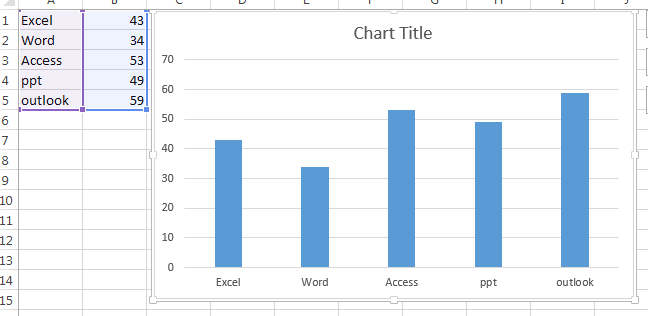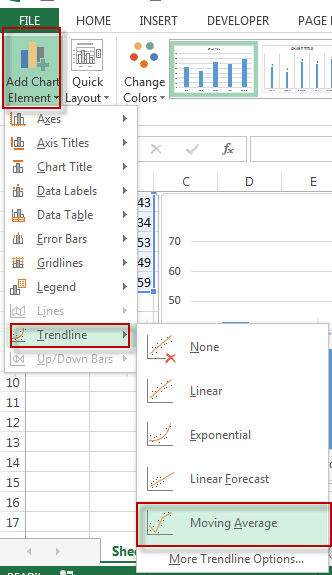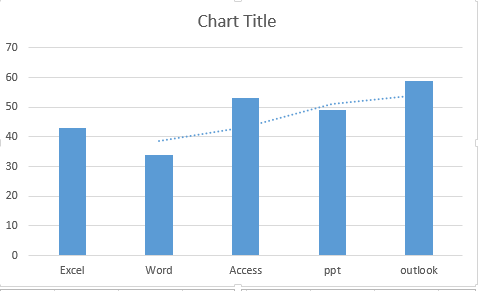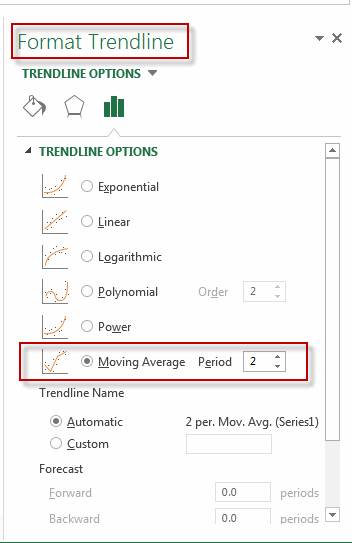Add a Moving Average Line to a Chart

This post will guide you how to add a moving average line to an Excel chart. How do I add moving average line in an excel chart.

A moving average is an average of the data in your table, and it will calculate over a defined period of time. So you can use the moving average linen to identify significant trends in the chart. And the moving average uses a specific number of data points to average them, and then use the average value as a point in the chart line.

Add a Moving Average Line to a Chart

The below steps will talk that how to add moving average line in an existing chart.

#1 select the column chart that you want to show the moving average line#2 go to DESIGN tab, click Add Chart Element command under Chart Layouts group, and click Trendline menu from the pop-up menu list, and then select moving average.#3 you will see that the moving average line is added in the current chart.#4 if you want to format the moving average line, for example, you want to change the period of the moving average line, you can double click on the moving average line in the chart, then the Format Trendline panel will appear, just click TrendLine Options to change the period.Related Posts

Filter Data by Column and Sort by Row

This post will guide you how to use the FILTER function to filter a value by column and then sort data by row in Microsoft Excel. You can use the following formula based on the SORT function in combination with ...

Filter And Transpose Data From Horizontal To Vertical

This post will show you how to use Filter function and in combination with Transpose function to filter data from horizontal and transpose data as vertical in Microsoft Excel. You can refer to the below general formula based on TRANSPOSE ...

VLOOKUP Formula | Faster Trick with 2 VLOOKUPS

This post will guide you how to use 2 VLOOKUPS function to looking up data entries from a given range of cells in Microsoft Excel. VLOOKUP with 2 lookups can be faster than a single VLOOKUP in certain scenarios. The ...

Extract Unique Items From A List

This post will guide you how to extract unique itmes from a given list in Microsoft Excel. How to create a newly formula to get unique values from a range cells in Excel. The unique list of items is the ...

Extract substring In Excel

This post will guide you how to use Excel's MID function is a quick and easy way to extract pieces from your text. Use the Excel formula to extract a substring with MID. Note: If you want to extract just ...

How to Use 3D SUM Multiple Worksheets

To sum a range of numbers is straightforward for most Excel users, but do you know how to establish a 3D reference to total the same range of numerous sheets. In this post, I will present the steps for this ...

Extract all the matches with helper Column

With Excel's powerful functions IF, INDEX, and MATCH, we can find exactly what you're looking for with a few clicks of the mouse. This step-by-step tutorial will show how easy it is to extract data using these tools and more! ...

If Cell is This Value or That Value

IF function is frequently used in Excel worksheet to return you expect “true value” or “false value” based on the result of logical test. If you want to see if a cell is A or B, and if one of ...

If Value is Greater Than A Certain Value

IF function is frequently used in Excel worksheet to return you expect “true value” or “false value” based on the logical test result. If you want to see if a value in one cell is greater than a specific value, ...

If Cell is Not Blank

IF function is frequently used in Excel worksheet to return you expect “true value” or “false value” based on the result of created logical test. If you want to see if a cell is blank or not, and leave some ...

Sidebar